## 15 Nov Ten topics to teach using Bar Models

Since the start of the school year, lots of primary teachers have been updating their schools’ mathematical calculations policies and discussing the pros and cons of potential core models. This got us thinking about which representations and classroom manipulatives are best for teaching each part of the maths curriculum.

Time and again Bar Models kept coming up – they’re such a useful resource to have in our teaching toolkit!

Here are our top 10 topics that we think you should be using Bar Models to teach:

1. Bridging between Concrete, Pictorial and Abstract

Because Bar Models closely resemble classroom manipulatives (such as dienes and blocks), they make the jump from using physical objects, to representing maths problems pictorially, far less daunting.

They are also incredibly flexible: to begin with we can incorporate images of objects or present the bar as a ruler with units of measurement, for example. Gradually we can remove the pictures and children will still understand that the numbers and length of the bars represent a physical value.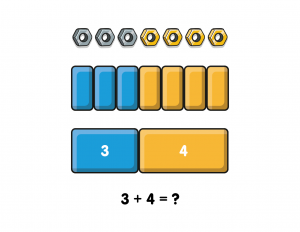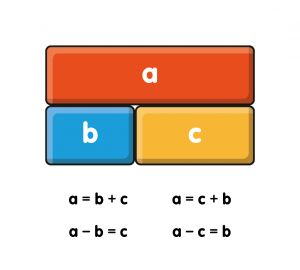2. Explaining the inverse relationship between addition and subtraction

Bar Models make solving missing number questions simple – if a child knows two of the values in a question, they can always calculate the other. This understanding also helps them recognise number bonds in different forms, such as when the “equals” sign is in a different position, giving them the confidence to tackle unfamiliar questions.

3. Proving Commutativity

Commutative law states that, in addition and multiplication sums, we can swap the two numbers around and the answer will still be the same (i.e. that “8 + 4” is equal to “4 + 8”). We can use bar models to prove this to the children, as they can see that the bars are the same length, whichever way around the parts are.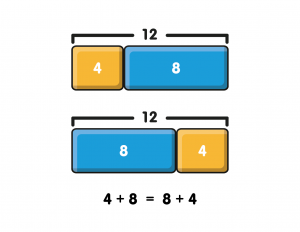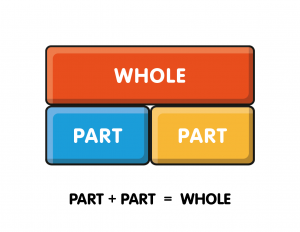Bar Models are particularly powerful at showing that when two (or more) numbers come together, they make another number. They are helpful at explaining that part + part = whole, and are a nice stepping stone onto the “cherry” part-whole model.

Rephrase questions involving Bar Models to make them less intimidating, such as asking how long the bar will be when another part is added onto it. (We can also use Bar Models to explore the inverse operation, by asking how long the bar would be if part was taken away, or how much of the bar would remain.)

Tip: Bar Models can be very effective when used in conjunction with number lines, as both representations present horizontally.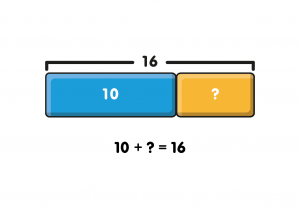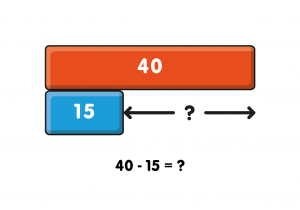6. Subtraction: Calculating the difference

As the Bar Model is a spatial representation, it enables children to visualise the physical difference between two values which, in turn, helps them work out how much they need to add onto the subtrahend bar in order to make it the same length as the minuend bar above. This can be done by counting up to the next multiple of ten, then by adding the tens, and then any ones.

7. Partitioning numbers in different ways

When it comes to partitioning numbers – especially into tens and ones – the Bar Model is an invaluable tool. Ask the children to draw how many different ways they could partition a two digit number into tens and ones. (They could use physical Dienes blocks for support.)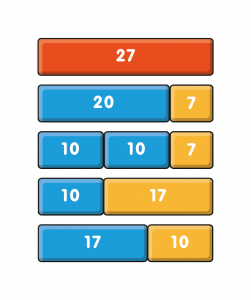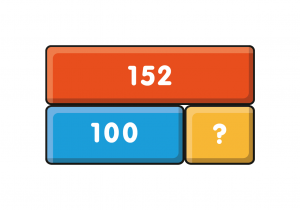8. Representing larger numbers

Unlike many other pictorial representations, where each “one” is represented by an individual object, Bar Models are able to efficiently represent three and four digit numbers. We can reuse this rare spatial representation throughout a child’s mathematical journey, helping them to notice patterns between topics in order to tackle increasingly complex questions.

9. Making comparisons (and tackling word problems)

By representing values spatially, Bar Models explicitly show the difference in length between certain values, proving whether one number is more or less than another.

Pupils can draw Bar Models to help them visualise word problems and explain their workings, right through to KS4 – especially for questions asking whether there is enough of something, often involving units of measurement or currency.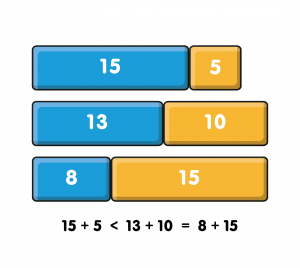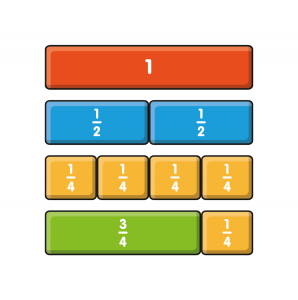10. Introducing fractions

By using a representation the children are already familiar with, we can make the jump to learning about fractions less daunting as children know they’ve seen something similar before.

Bar Models also encourage children to use the correct terminology when talking about fractions from the start. They immediately recognise that halves are two equal parts of a whole, and that quarters are four equal parts of a whole.

BONUS TIP: Show your pupils how to freehand draw Bar Models fairly accurately, so that they can use them to estimate answers to addition, subtraction and number bond questions. By helping children predict what the approximate value will be, Bar Models are really useful for supporting children to quickly check their work by flagging any obvious mistakes.